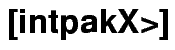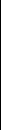Functional RangeReal Arithmetic Interval Type and construction/conversion functions   Arithmetic operations for intervals: &+, &-, &*, &/, also extended division   Auxiliary functions: midpoint, width, is_in, union, intersect...   Trigonometric functions: &sin, &cos, &tan, &arcsin, &arccos, &arctan, &sinh, &cosh, &tanh   Exponential function and logarithm   Square root, Square and Power functions   Interval Newton Method for continuously differentiable real functions (Example >>)   Range Enclosure for real-valued functions in one variable using three different evaluation methods (Example >>)   Range Enclosure for real-valued functions in two variables (Example >>) Complex Arithmetic Complex Disc Interval Type with plotting function   Arithmetic operations for disc intervals: &cadd, &csub, &cmult, &cdiv   Area optimal mutliplication and division: &cmult_opt, &cdiv_opt   Range Enclosure for Complex Polynomials using three different evaluation methods (Example >>)   Complex Exponential FunctionintpakX     Wiss. Rechnen / Softwaretechnologie     FB Mathematik     Bergische Universität Wuppertal